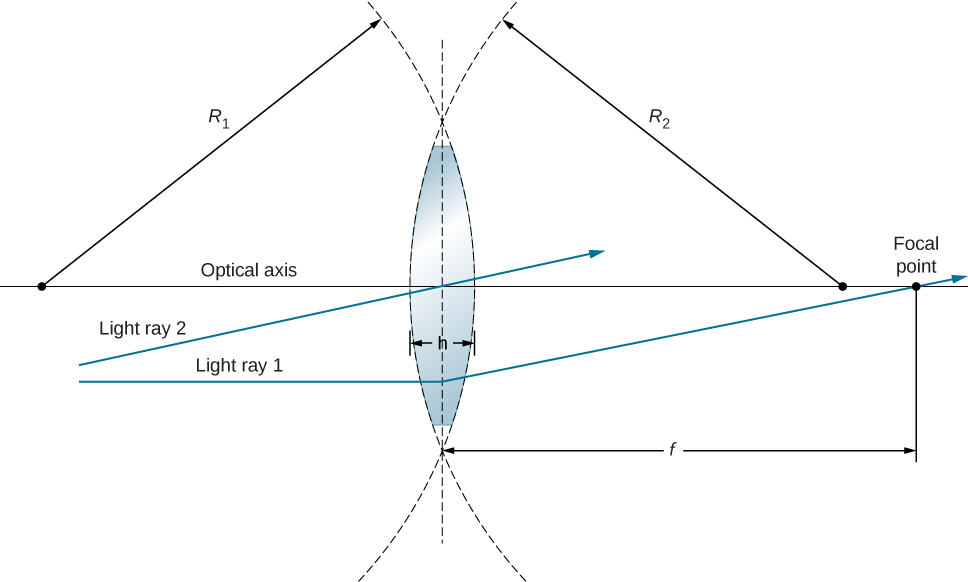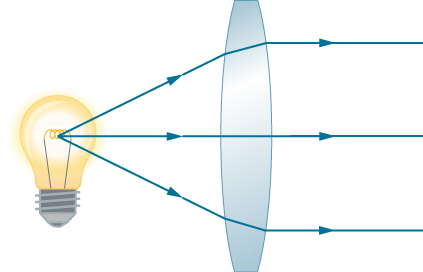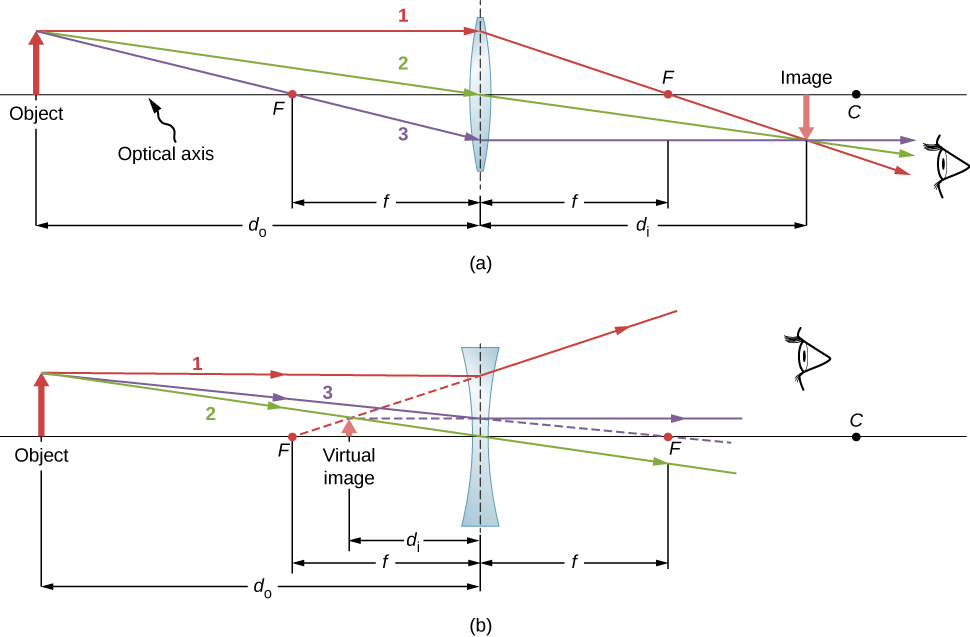# 2.4 Thin lenses  (Page 2/13)

 Page 2 / 13In the thin-lens approximation, the thickness d of the lens is much, much less than the radii R 1 and R 2 of curvature of the surfaces of the lens. Light rays are considered to bend at the center of the lens, such as light ray 1. Light ray 2 passes through the center of the lens and is undeviated in the thin-lens approximation.

As noted in the initial discussion of Snell’s law, the paths of light rays are exactly reversible. This means that the direction of the arrows could be reversed for all of the rays in [link] . For example, if a point-light source is placed at the focal point of a convex lens, as shown in [link] , parallel light rays emerge from the other side.A small light source, like a light bulb filament, placed at the focal point of a convex lens results in parallel rays of light emerging from the other side. The paths are exactly the reverse of those shown in [link] in converging and diverging lenses. This technique is used in lighthouses and sometimes in traffic lights to produce a directional beam of light from a source that emits light in all directions.

## Ray tracing and thin lenses

Ray tracing is the technique of determining or following (tracing) the paths taken by light rays.

Ray tracing for thin lenses is very similar to the technique we used with spherical mirrors. As for mirrors, ray tracing    can accurately describe the operation of a lens. The rules for ray tracing for thin lenses are similar to those of spherical mirrors:

1. A ray entering a converging lens parallel to the optical axis passes through the focal point on the other side of the lens (ray 1 in part (a) of [link] ). A ray entering a diverging lens parallel to the optical axis exits along the line that passes through the focal point on the same side of the lens (ray 1 in part (b) of the figure).
2. A ray passing through the center of either a converging or a diverging lens is not deviated (ray 2 in parts (a) and (b)).
3. For a converging lens, a ray that passes through the focal point exits the lens parallel to the optical axis (ray 3 in part (a)). For a diverging lens, a ray that approaches along the line that passes through the focal point on the opposite side exits the lens parallel to the axis (ray 3 in part (b)).Thin lenses have the same focal lengths on either side. (a) Parallel light rays entering a converging lens from the right cross at its focal point on the left. (b) Parallel light rays entering a diverging lens from the right seem to come from the focal point on the right.

Thin lenses work quite well for monochromatic light (i.e., light of a single wavelength). However, for light that contains several wavelengths (e.g., white light), the lenses work less well. The problem is that, as we learned in the previous chapter, the index of refraction of a material depends on the wavelength of light. This phenomenon is responsible for many colorful effects, such as rainbows. Unfortunately, this phenomenon also leads to aberrations in images formed by lenses. In particular, because the focal distance of the lens depends on the index of refraction, it also depends on the wavelength of the incident light. This means that light of different wavelengths will focus at different points, resulting is so-called “chromatic aberrations.” In particular, the edges of an image of a white object will become colored and blurred. Special lenses called doublets are capable of correcting chromatic aberrations . A doublet is formed by gluing together a converging lens and a diverging lens. The combined doublet lens produces significantly reduced chromatic aberrations.

what is bohrs model for hydrogen atom
hi
Tr
Hello
Youte
Hi
Nwangwu-ike
hi
Siddiquee
what is the value of speed of light
1.79×10_¹⁹ km per hour
Swagatika
what r dwarf planet
what is energy
কাজের একক কী
Jasim
কাজের একক কী
Jasim
friction ka direction Kaise pata karte hai
friction is always in the opposite of the direction of moving object
Punia
A twin paradox in the special theory of relativity arises due to.....? a) asymmetric of time only b) symmetric of time only c) only time
b) symmetric of time only
Swagatika
fundamental note of a vibrating string
every matter made up of particles and particles are also subdivided which are themselves subdivided and so on ,and the basic and smallest smallest smallest division is energy which vibrates to become particles and thats why particles have wave nature
Alvin
what are matter waves? Give some examples
according to de Broglie any matter particles by attaining the higher velocity as compared to light'ill show the wave nature and equation of wave will applicable on it but in practical life people see it is impossible however it is practicaly true and possible while looking at the earth matter at far
Manikant
a centeral part of theory of quantum mechanics example:just like a beam of light or a water wave
Swagatika
Mathematical expression of principle of relativity
given that the velocity v of wave depends on the tension f in the spring, it's length 'I' and it's mass 'm'. derive using dimension the equation of the wave
What is the importance of de-broglie's wavelength?
he related wave to matter
Zahid
at subatomic level wave and matter are associated. this refering to mass energy equivalence
Zahid
it is key of quantum
Manikant
how those weight effect a stable motion at equilibrium
how do I differentiate this equation- A sinwt with respect to t
just use the chain rule : let u =wt , the dy/dt = dy/du × du/dt : wA × cos(wt)
Jerry
I see my message got garbled , anyway use the chain rule with u= wt , etc...
Jerry
de broglie wave equation
vy beautiful equation
chandrasekhar
what is electro statics
when you consider systems consisting of fixed charges
SherlyBy JavaChamp TeamBy MldelatteBy Dan ArielyBy OpenStaxBy Madison ChristianBy OpenStaxBy John GabrieliBy OpenStaxBy David MartinBy Richley Crapo